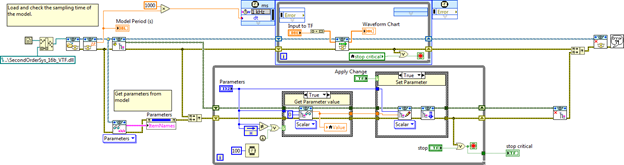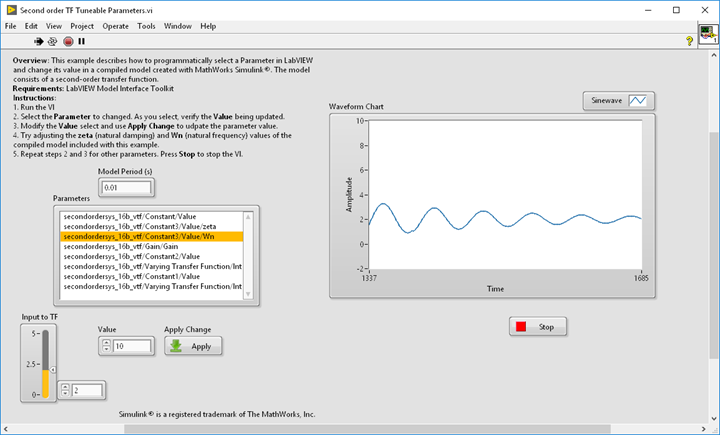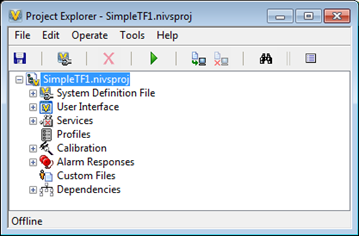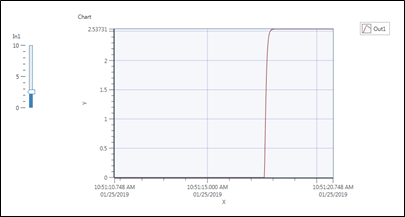# Example Code

## Products and Environment

This section reflects the products and operating system used to create the example.

• LabVIEW
• Other
• Veristand

## Overview

You can use LabVIEW and VeriStand to run compiled models (DLLs) created using MathWorks Simulink® and Simulink® CoderTM.  The models in these examples are based on continuous time, second-order transfer functions and include fixed-step numerical solvers from the MathWorks.  A fixed-step solver (ode 1) was chosen in Simulink so that these models can also run on real-time targets.  One of the compiled Simulink models includes a MATLAB® function block to illustrate an option for bringing your compiled MATLAB code to NI real-time targets.

This interfacing approach between compiled models from Simulink and LabVIEW or VeriStand can be used to implement algorithms, filters, control systems, estimators, and real-time models in your applications using NI hardware. You can take these examples and modify them on your computer and real-time hardware to use your own DLLs from Simulink Coder.

## Description

The table below shows the files included with these examples (in two attached zip files).  Each of the rows in the table corresponds to a LabVIEW VI or VeriStand project calling a specific Simulink model DLL.  You need to install the LabVIEW VIs and DLLs together in the same folder on your hard drive. The last row in the table corresponds to a VeriStand project which calls one of the Simulink model DLLs. You need to install the files in a folder in this location:  ..Documents\National Instruments\NI VeriStand 201x\Projects

 Example LabVIEW VIs / VeriStand Projects Compiled Simulink Models Model Description 1 Second order TF Simple Load and Run Model.vi SecondOrderSys_16b_TF.dll Damping ratio and natural frequency both set to 1 (critically damped) Model period = 0.2 sec 2 Second order TF Tuneable Parameters.vi SecondOrderSys_16b_VTF.dll Variable damping ratio and natural frequency Model period = 0.01 sec 3 Second order TF with MATLAB.vi SecondOrderSys_16b_VTFwNoise.dll MATLAB function block added to output of transfer function Model period = 0.01 sec 4 SimpleTF1 VeriStand project SecondOrderSys_16b_TF.dll Damping ratio and natural frequency both set to 1 (critically damped) Model period = 0.2 sec

Notes:

• The compiled Simulink models will run on both Windows computers and on NI real-time targets with the Phar Lap ETS real-time operating system (such as embedded PXI systems). They can be called by either LabVIEW or VeriStand.
• If you want to use LabVIEW but not VeriStand, you configure the NI VeriStand installer (see figure below) to install just the NI VeriStand Model Framework and the LabVIEW Model Interface Toolkit.
• Because the Simulink models are compiled using Simulink Coder, you do not need to have MATLAB or Simulink installed on your Windows or real-time machine to run these demonstrations.
For myRIO or CompactRIO targets with the NI Linux Real-Time OS, you will need to compile Simulink models using Simulink Coder and VeriStand with NI Linux Real-Time selected as the target. You also need to install LabVIEW myRIO Toolkit or the CompactRIO driver on your development computer.SecondOrderSys_16b_TF.dllSecondOrderSys_16b_VTFwNoise.dll:

(SecondOrderSys_16b_VTF.dll is similar but does not have the MATLAB function block)

##The three compiled Simulink models were created by The MathWorks, Inc. using Simulink R2016b and then compiled with:

• NI VeriStand Model Framework
• Microsoft Visual Studio 2010

The models are provided with a software license described in the included license.txt file.

## Hardware and Software Requirements

Required software for Windows:

• LabVIEW 2018 (32-bit)
• LabVIEW 2018 Model Interface Toolkit (installed using NI VeriStand installer)
• VeriStand 2017 or 2018 (required for VeriStand demo only)

No hardware required.

## Steps to Implement or Execute Code

Example 1:  Open and run the Second order TF Simple Load and Run Model.vi. This VI will load the SecondOrderSys_16b_TF.dll, get the model period, and then run the model. Adjust the Offset control on the front panel to provide a step input to the dynamic system.Example 2:  Open and run the Second order TF Tuneable Parameters.vi. This VI will load the SecondOrderSys_16b_VTF.dll, get the model period, and run the model while also allowing you to change model parameters. Adjust the Input to TF control on the front panel to provide a step input to the system. Additionally, you can select the zeta (damping ratio) and Wn (natural frequency) parameters, change the parameter values, and then hit the Apply button for each. The front panel screenshot below shows the response after the damping ratio was changed to 0.1 and the natural frequency was changed to 10 rad/sec.Example 3:  Open and run the Second order TF with MATLAB.vi. This VI will load the SecondOrderSys_16b_VTFwNoise.dll, get the model period, and run the model. Adjust the Offset control on the front panel to provide a step input to the system. The model includes the same second order transfer function as in demonstration 2, but we are leaving the damping ratio and natural frequency parameters set to their default values. This model includes a MATLAB function block added to the output of the transfer function. The MATLAB function block takes the output of the Simulink transfer function, adds random noise, and then multiplies it by two:  y = 2*(u + 0.1*randn);  Note that the final value of the system response is two times the step input to the system and the response also includes random noise.Example 4: Open and run the SimpleTF1 VeriStand project.  You can change In1, the input value to the compiled model, and observe the response on the chart.